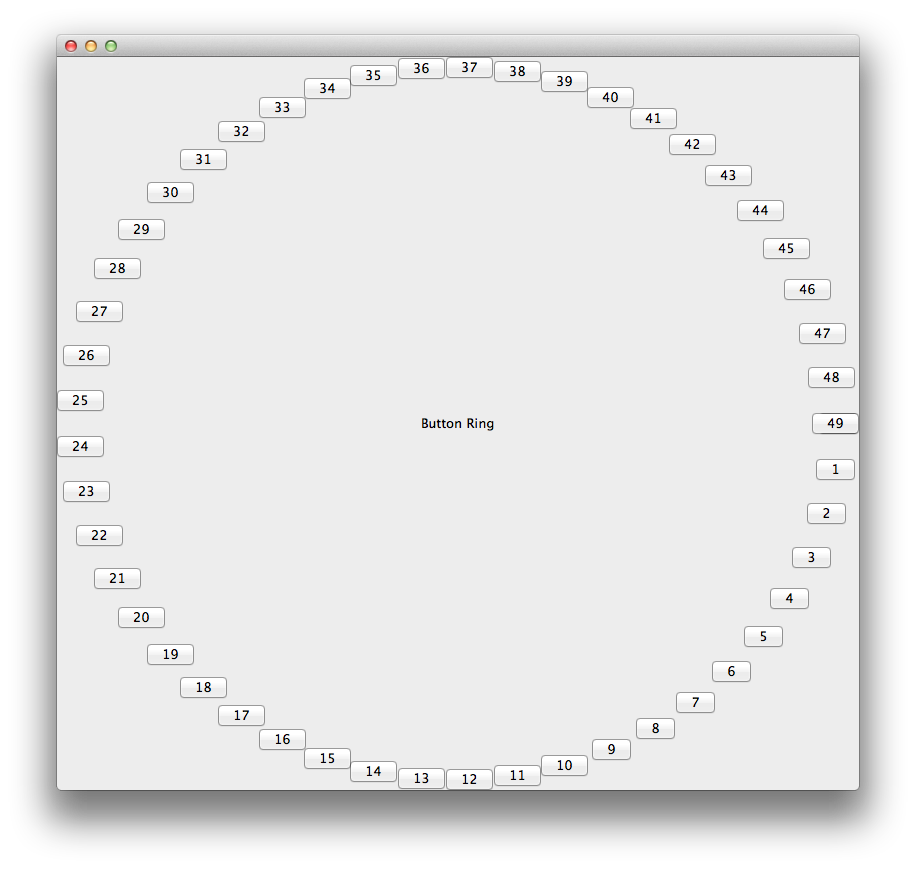# ButtonRing Example¶

button_ring.enaml

```#------------------------------------------------------------------------------
#  Copyright (c) 2012, Enthought, Inc.
#------------------------------------------------------------------------------
""" An example of a layout which cannot be achieved with typical layout
managers.

This example creates a ring of 50 `PushButton` widgets with a `Label` in
the center. The constraints shown here are generally silly in that the
resulting layout is more-or-less useless. Nevertheless, it serves well to
demonstrate the power and flexibility of constraints-based layout.

Note that the 'gen_constraints' function is only called once, not on
every resize as may be expected when laying out widgets manually.

Note that this example also demonstrates that constraints may be defined
on any subclass of `ConstraintsWidget`. They need not be confined to a
`Container`.

"""
import numpy as np
from enaml.widgets.api import MainWindow, Container, PushButton, Label
from enaml.widgets.include import Include

def gen_constraints(comps, top, left, width, height):
""" A helper function which generates radial constraints.

Parameters
----------
comps : list
A list of ConstraintWidget instances which should be arrange
radially over an intervale of 2-Pi.

top : ConstraintVariable
The variable representing the top of the layout area.

left : ConstraintVariable
The variable representing the left of the layout area.

width : ConstraintVariable
The variable representing the width of the layout area.

height : ConstraintVariable
The variable representing the height of the layout area.

Returns
-------
result : list
The list of primitive constraints which arrange the given
components radially in the layout area.

"""
res = []
nitems = len(comps)
rads = np.linspace(0, 2 * np.pi, nitems)
x_coeffs = (np.cos(rads) + 1.0) / 2.0
y_coeffs = (np.sin(rads) + 1.0) / 2.0
for comp, x_coeff, y_coeff in zip(comps, x_coeffs, y_coeffs):
res.extend([
comp.left == (left + x_coeff * (width - comp.width)),
comp.top == (top + y_coeff * (height - comp.height)),
])
return res

enamldef SimpleButton(PushButton):
""" A custom `PushButton` for use in the button ring example.

The width and height of this `PushButton` is fixed to its size hint.
This is required since no other constraints are placed on the width
of the button in this example. If these constraints were not added,
then the constraints solver would error with an unbounded system,
indicating an underconstrained system.

"""
hug_width = 'required'
hug_height = 'required'
clicked :: print '%s clicked' % text

enamldef Main(MainWindow):
Container:
id: cntr
constraints << gen_constraints(
inc.objects, top, left, width, height,
) + [width >= 200, height >= 200]
Include:
id: inc
objects = [SimpleButton(text=str(i)) for i in range(50)]
Label:
id: lbl
# Constraints can also be directly defined on a component.
# This is provided as a convenience where it makes the code
# clearer and easier to understand. It makes no difference
# if the constraints are defined here, or on the Container.
constraints = [
v_center == cntr.v_center,
h_center == cntr.h_center,
]
text = 'Button Ring'
font = 'arial 12 bold'

```
`\$ enaml-run button_ring.enaml`